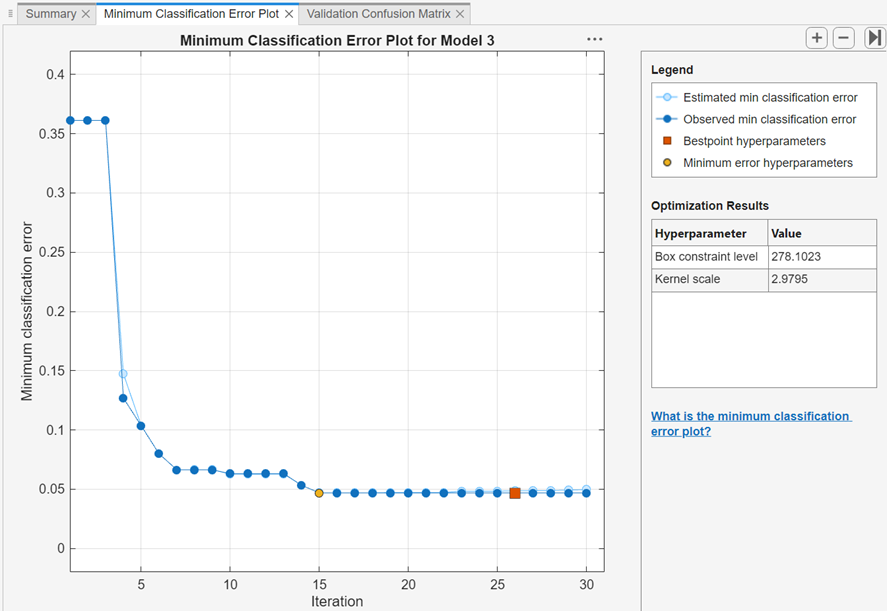## Train Classifier Using Hyperparameter Optimization in Classification Learner App

This example shows how to tune hyperparameters of a classification support vector machine (SVM) model by using hyperparameter optimization in the Classification Learner app. Compare the test set performance of the trained optimizable SVM to that of the best-performing preset SVM model.

1. In the MATLAB® Command Window, load the `ionosphere` data set, and create a table containing the data. Separate the table into training and test sets.

```load ionosphere tbl = array2table(X); tbl.Y = Y; rng('default') % For reproducibility of the data split partition = cvpartition(Y,'Holdout',0.15); idxTrain = training(partition); % Indices for the training set tblTrain = tbl(idxTrain,:); tblTest = tbl(~idxTrain,:);```
2. Open Classification Learner. Click the Apps tab, and then click the arrow at the right of the Apps section to open the apps gallery. In the Machine Learning and Deep Learning group, click Classification Learner.

3. On the Classification Learner tab, in the File section, select New Session > From Workspace.

4. In the New Session dialog box, select the `tblTrain` table from the Data Set Variable list.

As shown in the dialog box, the app selects the response and predictor variables. The default response variable is `Y`. The default validation option is 5-fold cross-validation, to protect against overfitting. For this example, do not change the default settings.5. To accept the default options and continue, click .

6. Train all preset SVM models. On the Classification Learner tab, in the Model Type section, click the arrow to open the gallery. In the Support Vector Machines group, click . In the Training section, click . The app trains one of each SVM model type and displays the models in the History list.

Tip

If you have Parallel Computing Toolbox™, the Opening Pool dialog box opens the first time you click (or when you click again after an extended period of time). The dialog box remains open while the app opens a parallel pool of workers. During this time, you cannot interact with the software. After the pool opens, you can train multiple models simultaneously and continue working.Note

Validation introduces some randomness into the results. Your model validation results can vary from the results shown in this example.

7. Select an optimizable SVM model to train. On the Classification Learner tab, in the Model Type section, click the arrow to open the gallery. In the Support Vector Machines group, click . The app disables the button when you select an optimizable model.

8. Select the model hyperparameters to optimize. In the Model Type section, select Advanced > Advanced. The app opens a dialog box in which you can select Optimize check boxes for the hyperparameters that you want to optimize. By default, all the check boxes for the available hyperparameters are selected. For this example, clear the Optimize check boxes for Kernel function and Standardize data. By default, the app disables the Optimize check box for Kernel scale whenever the kernel function has a fixed value other than `Gaussian`. Select a `Gaussian` kernel function, and select the Optimize check box for Kernel scale.9. In the Training section, click .

10. The app displays a Minimum Classification Error Plot as it runs the optimization process. At each iteration, the app tries a different combination of hyperparameter values and updates the plot with the minimum validation classification error observed up to that iteration, indicated in dark blue. When the app completes the optimization process, it selects the set of optimized hyperparameters, indicated by a red square. For more information, see Minimum Classification Error Plot.

The app lists the optimized hyperparameters in both the upper right of the plot and the Optimized Hyperparameters section of the Current Model pane.Note

In general, the optimization results are not reproducible.

11. Compare the trained preset SVM models to the trained optimizable model. In the History list, the app highlights the highest validation Accuracy by outlining it in a box. In this example, the trained optimizable SVM model outperforms the six preset models.

A trained optimizable model does not always have a higher accuracy than the trained preset models. If a trained optimizable model does not perform well, you can try to get better results by running the optimization for longer. In the Model Type section, select . In the dialog box, increase the Iterations value. For example, you can double-click the default value of `30` and enter a value of `60`.

12. Because hyperparameter tuning often leads to overfitted models, check the test set performance of the SVM model with the optimized hyperparameters and compare it to the performance of the best preset SVM model. Begin by exporting the two models to the MATLAB workspace.

• In the History list, select the Medium Gaussian SVM model. On the Classification Learner tab, in the Export section, select . In the dialog box, name the model `gaussianSVM`.

• In the History list, select the Optimizable SVM model. On the Classification Learner tab, in the Export section, select . In the dialog box, name the model `optimizableSVM`.

13. Compute the accuracy of the two models on the `tblTest` data. In the MATLAB Command Window, use the `predictFcn` function in each exported model structure to predict the response values of the test set data. Then, use confusion matrices to visualize the results. Compute and compare the accuracy values for the models on the test set data.

```testY = tblTest.Y; labels = gaussianSVM.predictFcn(tblTest); figure cm = confusionchart(testY,labels); title('Preset Model Results') optLabels = optimizableSVM.predictFcn(tblTest); figure optcm = confusionchart(testY,optLabels); title('Optimizable Model Results') cmvalues = cm.NormalizedValues; optcmvalues = optcm.NormalizedValues; presetAccuracy = sum(diag(cmvalues))/sum(cmvalues,'all')*100 optAccuracy = sum(diag(optcmvalues))/sum(optcmvalues,'all')*100 ```
```presetAccuracy = 92.3077 optAccuracy = 88.4615```In this example, the trained optimizable SVM model does not perform as well as the trained preset model on the test set data.